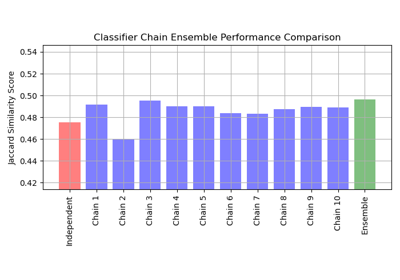# sklearn.metrics.jaccard_score¶

sklearn.metrics.jaccard_score(y_true, y_pred, *, labels=None, pos_label=1, average='binary', sample_weight=None)[source]

Jaccard similarity coefficient score

The Jaccard index , or Jaccard similarity coefficient, defined as the size of the intersection divided by the size of the union of two label sets, is used to compare set of predicted labels for a sample to the corresponding set of labels in y_true.

Read more in the User Guide.

Parameters
y_true1d array-like, or label indicator array / sparse matrix

Ground truth (correct) labels.

y_pred1d array-like, or label indicator array / sparse matrix

Predicted labels, as returned by a classifier.

labelslist, optional

The set of labels to include when average != 'binary', and their order if average is None. Labels present in the data can be excluded, for example to calculate a multiclass average ignoring a majority negative class, while labels not present in the data will result in 0 components in a macro average. For multilabel targets, labels are column indices. By default, all labels in y_true and y_pred are used in sorted order.

pos_labelstr or int, 1 by default

The class to report if average='binary' and the data is binary. If the data are multiclass or multilabel, this will be ignored; setting labels=[pos_label] and average != 'binary' will report scores for that label only.

averagestring, [None, ‘binary’ (default), ‘micro’, ‘macro’, ‘samples’, ‘weighted’]

If None, the scores for each class are returned. Otherwise, this determines the type of averaging performed on the data:

'binary':

Only report results for the class specified by pos_label. This is applicable only if targets (y_{true,pred}) are binary.

'micro':

Calculate metrics globally by counting the total true positives, false negatives and false positives.

'macro':

Calculate metrics for each label, and find their unweighted mean. This does not take label imbalance into account.

'weighted':

Calculate metrics for each label, and find their average, weighted by support (the number of true instances for each label). This alters ‘macro’ to account for label imbalance.

'samples':

Calculate metrics for each instance, and find their average (only meaningful for multilabel classification).

sample_weightarray-like of shape (n_samples,), default=None

Sample weights.

Returns
scorefloat (if average is not None) or array of floats, shape = [n_unique_labels]

Notes

jaccard_score may be a poor metric if there are no positives for some samples or classes. Jaccard is undefined if there are no true or predicted labels, and our implementation will return a score of 0 with a warning.

References

1

Wikipedia entry for the Jaccard index

Examples

>>> import numpy as np
>>> from sklearn.metrics import jaccard_score
>>> y_true = np.array([[0, 1, 1],
...                    [1, 1, 0]])
>>> y_pred = np.array([[1, 1, 1],
...                    [1, 0, 0]])


In the binary case:

>>> jaccard_score(y_true, y_pred)
0.6666...


In the multilabel case:

>>> jaccard_score(y_true, y_pred, average='samples')
0.5833...
>>> jaccard_score(y_true, y_pred, average='macro')
0.6666...
>>> jaccard_score(y_true, y_pred, average=None)
array([0.5, 0.5, 1. ])


In the multiclass case:

>>> y_pred = [0, 2, 1, 2]
>>> y_true = [0, 1, 2, 2]
>>> jaccard_score(y_true, y_pred, average=None)
array([1. , 0. , 0.33...])


## Examples using sklearn.metrics.jaccard_score¶